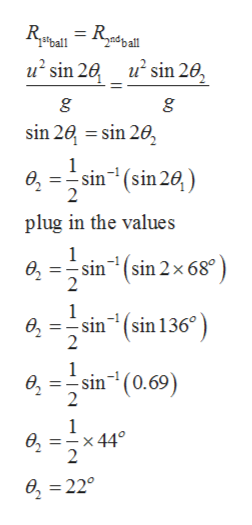# One strategy in a snowball fight is to throw a snowball at a high angle over level ground. While your opponent is watching this first snowball, you throw a second snowball at a low angle and time it to arrive at the same time as the first. Assume both snowballs are thrown with the same initial speed 20.5 m/s. The first snowball is thrown at an angle of 68◦ above the horizontal. At what angle should you throw the second snowball to make it hit the same point as the first? The acceleration of gravity is 9.8 m/s 2 . Answer in units of ◦ .

Question

One strategy in a snowball fight is to throw a snowball at a high angle over level ground. While your opponent is watching this first snowball, you throw a second snowball at a low angle and time it to arrive at the same time as the first. Assume both snowballs are thrown with the same initial speed 20.5 m/s. The first snowball is thrown at an angle of 68◦ above the horizontal. At what angle should you throw the second snowball to make it hit the same point as the first? The acceleration of gravity is 9.8 m/s 2 . Answer in units of ◦ .

check_circle

Step 1

Given:

For first ball, θ1 = 68ο

initial velocity for both the balls, u = 20.5 m/s

for second ball, θ2 = ?

Step 2

since distance covered by both the balls is same.

Therefore,...help_outlineImage Transcriptionclose2ndball 1stball u2 sin 2 u sin 20 sin 20 sin 202 1 e, =sin(sin 20,) 2 plug in the values '(sin 2x 68 2 sin 1 - sin ө, 1(sin 136° 1 sin(0.69) в, 2 1 в, Ex 44° 2 e22° fullscreen

### Want to see the full answer?

See Solution

#### Want to see this answer and more?

Solutions are written by subject experts who are available 24/7. Questions are typically answered within 1 hour.*

See Solution
*Response times may vary by subject and question.
Tagged in

### Kinematics